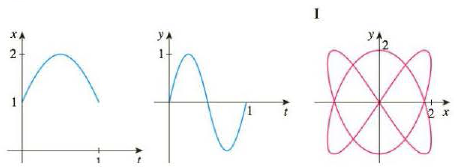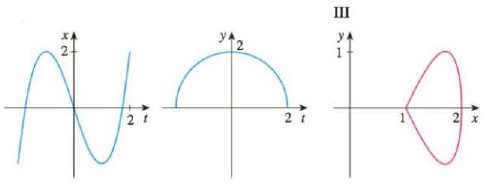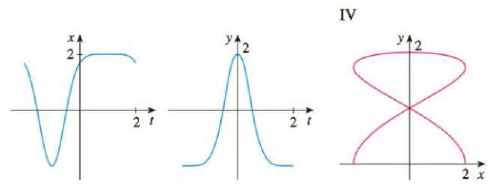Chapter 10.1, Problem 24E

Chapter
Section
Textbook Problem

Match the graphs of the parametric equations x = f(t) and y = g(t) in (a)–(d) with the parametric curves labeled I–IV. Give reasons for your choices. (a)(b)(c)(d)To determine

To match: The parametric equations x=f(t) and y=g(t) shown in graphs (a) to (d) with the parametric curves labeled I to IV.

Explanation

The parametric equations x=f(t) and y=g(t) shown in graph (a) matches with the parametric curve labeled III.

The parametric equations x=f(t) and y=g(t) shown in graph (b) matches with the parametric curve labeled I.

The parametric equations x=f(t) and y=g(t) shown in graph (c) matches with the parametric curve labeled IV.

The parametric equations x=f(t) and y=g(t) shown in graph (d) matches with the parametric curve labeled II.

Given:

The parametric equation for the variable x is as follows.

x=f(t)

The parametric equation for the variable y is as follows.

y=g(t)

Calculation:

Graph (a):

From graph (a), it is observed that the parameter x varies from (1x2) at the interval of (0t1) and parameter y varies from (1y1) at the interval of (0t1)

Plot the parametric curve for which the parameter x varies from (1x2) and y varies from (1y1) as shown below in Figure 1.

The parametric curve shown in Figure 1 resembles the parametric curve shown in graph–III. Therefore, the parametric equations x=f(t) and y=g(t) shown in graph (a) matches with the parametric curve labeled III.

Graph (b):

From graph (b), it is observed that the parameter x varies four times periodically from (2x2) at the interval of (0t1) and parameter y also varies six times periodically from (2y2) at the interval of (0t1) .

Plot the parametric curve for which the parameter x varies from (2x2) and y varies from (2y2) as shown below in Figure 2

Still sussing out bartleby?

Check out a sample textbook solution.

See a sample solution

The Solution to Your Study Problems

Bartleby provides explanations to thousands of textbook problems written by our experts, many with advanced degrees!

Get Started

Find more solutions based on key concepts